#Calculus Worksheets

## Indefinite Integration Worksheets

Here is a graphic preview for all of the Indefinite Integration for Calculus Worksheets. You can select different variables to customize these Indefinite Integration for Calculus Worksheets for your needs. The Indefinite Integration for Calculus Worksheets are randomly created and will never repeat so you have an endless supply of quality Indefinite Integration for Calculus Worksheets to use in the classroom or at home. We have exponential and trigonometric integration, power rule, substitution, and integration by parts worksheets.

Our Indefinite Integration for Calculus Worksheets are free to download, easy to use, and very flexible.

These Indefinite Integration for Calculus Worksheets are a good resource for students in high school.

Click here for a Detailed Description of all the Indefinite Integration for Calculus Worksheets.

## Quick Link for All Indefinite Integration for Calculus Worksheets

Click the image to be taken to that Indefinite Integration for Calculus Worksheets.

##### Power Rule Worksheets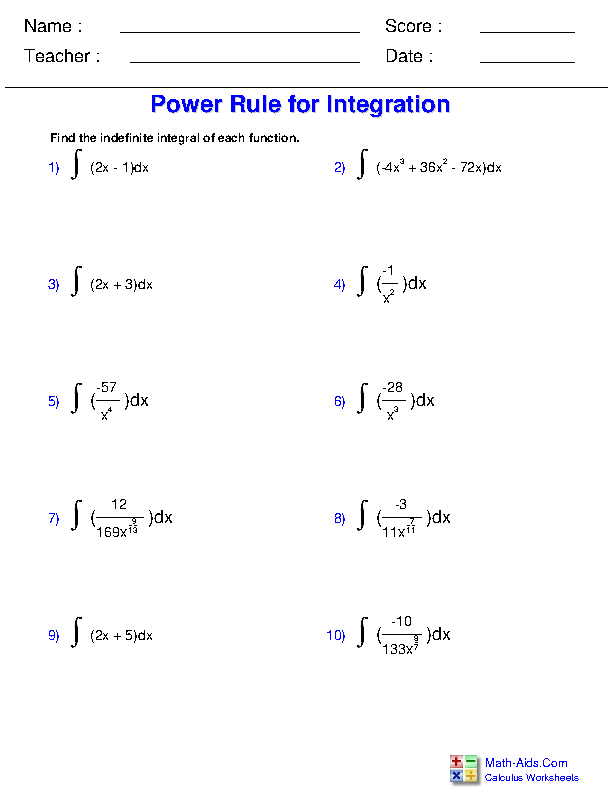##### Logarithmic and Exponential Integrals Worksheets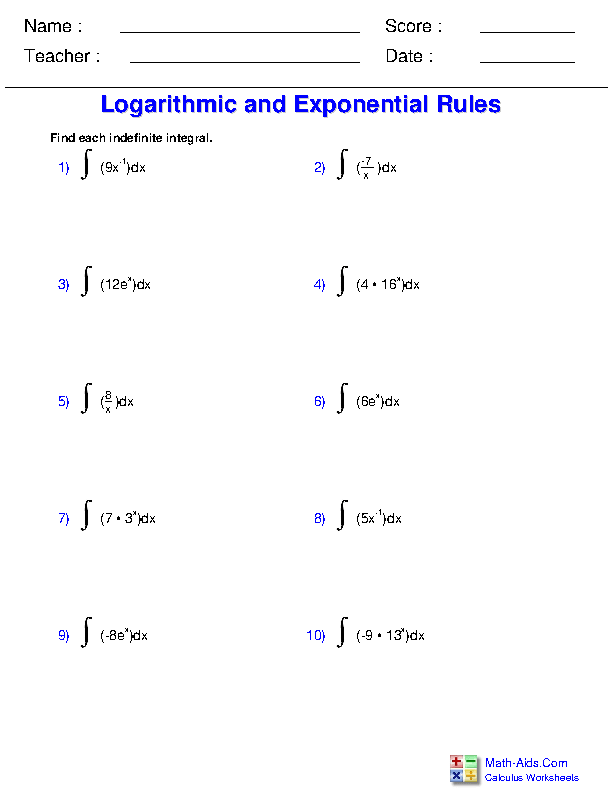##### Trigonometric Integration Worksheets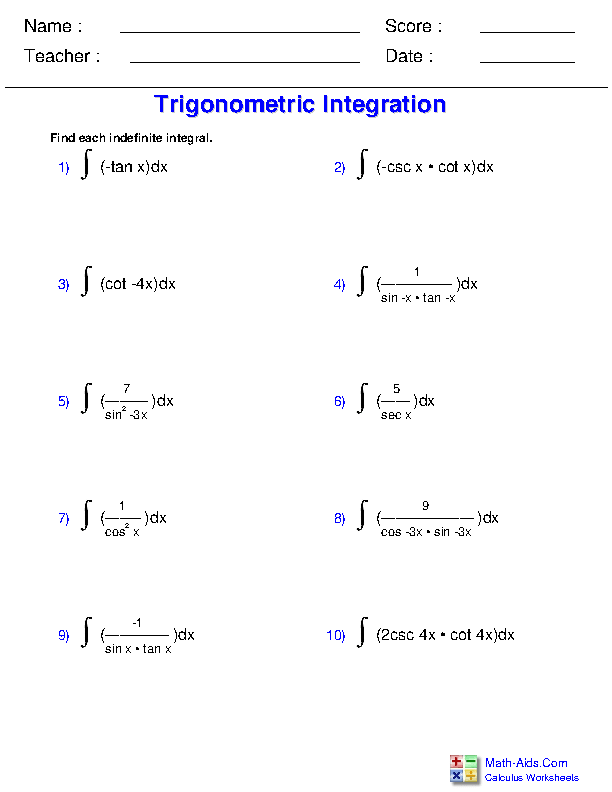##### Inverse Trigonometric Function Integrals Worksheets##### Substitution with the Power Rule Worksheets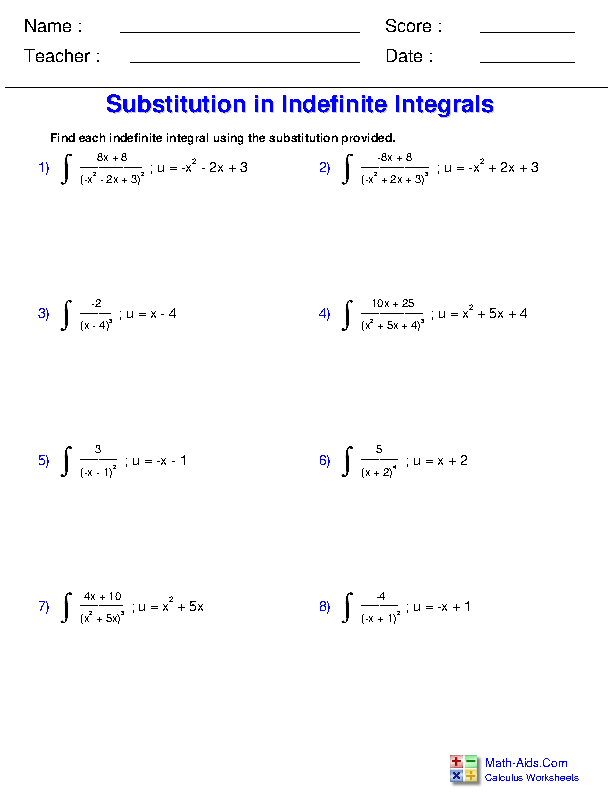##### Substitution with Logarithmic and Exponential Functions Worksheets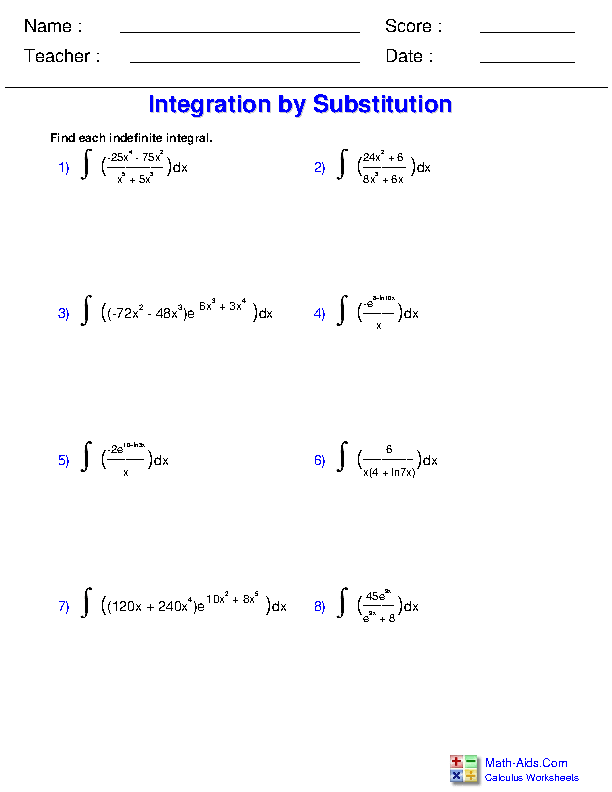##### Substitution with Trigonometric Functions Worksheets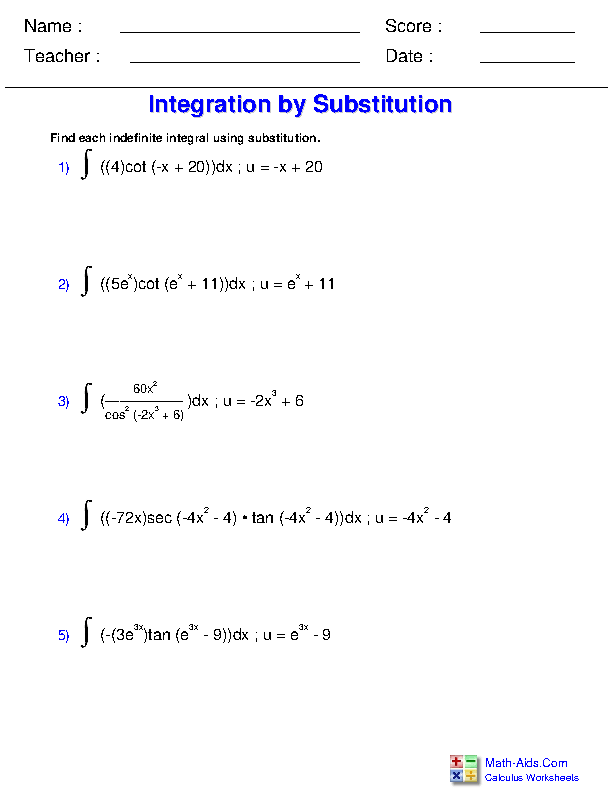##### Substitution with Inverse Trigonometric Functions Worksheets##### Integration by Parts Worksheets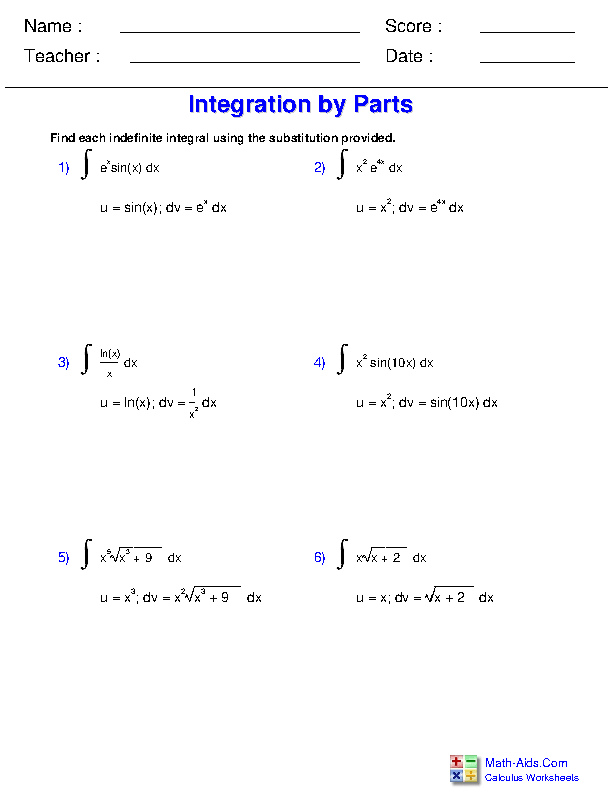Recommended Videos

## Detailed Description for All Indefinite Integration for Calculus Worksheets

Power Rule Worksheets
This Calculus - Indefinite Integration Worksheet will produce problems that involve finding an indefinite integral using the power rule.

Logarithmic and Exponential Integrals Worksheets
This Calculus - Indefinite Integration Worksheet will produce problems that involve using the logarithmic and exponential rules to find indefinite integrals.

Trigonometric Integration Worksheets
This Calculus - Indefinite Integration Worksheet will produce problems that involve using trigonometric function rules to solve indefinite integrals.

Inverse Trigonometric Function Integrals Worksheets
This Calculus - Indefinite Integration Worksheet will produce problems that involve using inverse trigonometric function rules to solve indefinite integrals.

Substitution with the Power Rule Worksheets
This Calculus - Indefinite Integration Worksheet will produce problems that involve using substitution to solve indefinite integrals using the power rule.

Substitution with Logarithmic and Exponential Functions Worksheets
This Calculus - Indefinite Integration Worksheet will produce problems that involve integrating logarithmic or exponential functions using substitution.

Substitution with Trigonometric Functions Worksheets
This Calculus - Indefinite Integration Worksheet will produce problems that involve using substitution to find the integrals of trigonometric functions.

Integration by Parts Worksheets
This Calculus - Indefinite Integration Worksheet will produce problems that involve solving indefinite integrals by using integration by parts.

Substitution with Inverse Trigonometric Functions Worksheets
This Calculus - Indefinite Integration Worksheet will produce problems that involve integrating inverse trigonometric functions using substitution.